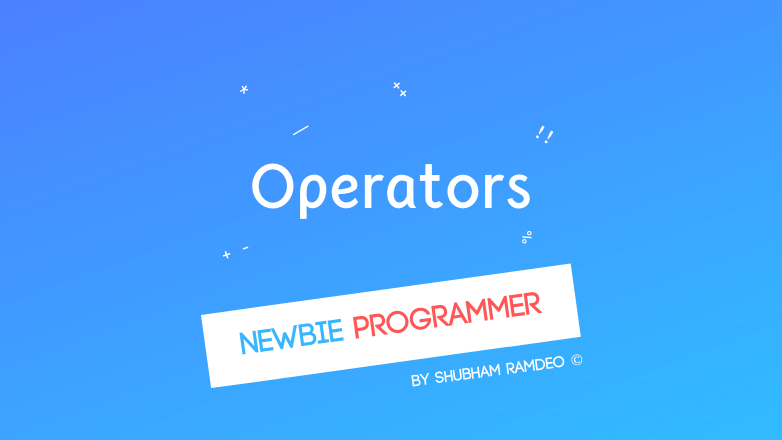# Operators Introduction

PUBLISHED Aug 17th, 2015

It’s the operators which really help computer program to decide and act !Starting here with a new Topic, Operators, Welcome to the Newbie Programmer Series. With the last part we have finished the Loops. Now before we go advanced, first we have to learn their correct usage. So If you are new to this series, please read out all the previous part from the Index (here).

But what is an operator ? This post is gonna be a Welcome-to / theoretical. Also if I put everything in one page, it will become hard to understand. Therefore I have divided this topic into parts.

Operators are the mathematical symbols that follows some operations. That mean each such symbol provides some sort of command or mainly a logic to make the program flow in the way we want. For an example, 3 + 4 has the “  + “ operator to perform the “addition” operation.  Our programming language, C, is rich in such operators !

### There are following types of operators that we are gonna learn:

1. Arithmetic Operators
2. Relational Operators
3. Logical Operators
4. Assignment Operators
5. Tricks and tips !

### Before learning the operators, we have to learn their Operations.

That’s the reason why I am dividing this into parts. Actually each of the above parts have different concepts connected to them. We may understand Arithmetic operators easily, simply + -  etc but for others, we have to study them separately. Specially the Bitwise Operators. You will have to first understand the computer’s zeros and ones to use this one.

Yes we will be learning machine’s language a bit. Or we may discuss the advanced topics later.

### Programming is almost impossible without operators

Can you imagine if a programming language, which cannot add two numbers, which cannot compare which is bigger between 2 and 5, which cannot check if X and Y are equal etc ? Not possible right ! I think operators are the power house of a language and C has plenty of useful operators.

### Most of the mistakes in a program is due to incorrect use of operators

People usually make mistakes such as x = y  and x == y. Actually “=” and “==” are very different. Also what will computer do if you say “ 2 + 7 / 6 “ will you first add  2 and 7 or first divide 7 by 6 ? Programmers knowingly “miss” such things and waste a lot of time finding errors. This is why I am forcing too much on operators because its only the incorrect use of operators that make programming ugly. But we will learn each of them separately. So as to understand their use deeply.

Stay connected !

#### You might also like:

(prev)
(next)

(rand) Is it possible to predict future?
(rand) Define Preprocessor
(rand) What are RGB and hexadecimal color codes? How colors are coded in computers?